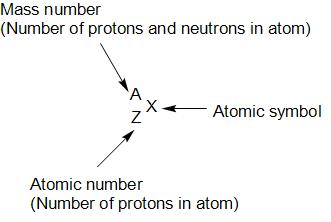$\left( {}_{32}G{{e}^{76}},{}_{34}S{{e}^{76}} \right) and \left( {}_{14}S{{i}^{30}},{}_{16}{{S}^{32}} \right)$ and are examples of:A. isotopes and isobarsB. isobars and isotonesC. isotones and isotopesD. isobars and isotopesVerified
141.3k+ views
Hint: In nuclear chemistry, the nuclides are classified based on the number of protons and neutrons of the nuclei as isotopes, isotones and isobars. We represent nuclide by:- Let’s discuss about $\left( {}_{32}G{{e}^{76}},{}_{34}S{{e}^{76}} \right)$ first:
- Let’s discuss about $\left( {}_{14}S{{i}^{30}},{}_{16}{{S}^{32}} \right)$: